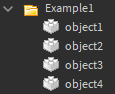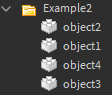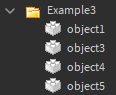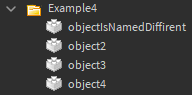# Creating objects sorting algorithm

I want to sort objects (instances) inside other instances.We have folder `Example1` and `objectX`s, I can get `X` by using

``````string.gsub(child.Name, "object", "")
``````

and then I would like to save it to the table. Like this below:

``````{
['1'] = workspace.Example1.object1,
['2'] = workspace.Example1.object2,
['3'] = workspace.Example1.object3,
['4'] = workspace.Example1.object4,
}
``````

now, I don’t care if order of them. They can be like thatbut, when we will have for example `1`, `3` and so on it will raise an error because we don’t have `2`.or when one of the objects is not named like a patternDoes someone have any idea about that?

Thank you!

1. Store the Instances in a table and set their parents to `nil`.
2. Sort the instances in the table by their names.
3. Iterate through the table and set the parent of each Instance to the target

I think you didnt understand me. I dont want to move them anywhere. Just check if Objects in Folder (in above example) are sorted so `object1` exist, `object2` exist and so on

Oh ok, I believe that you get the exact order if you just get the children with `Instance:GetChildren()`. Then you can evaluate the resulting table (see @nicemike40’s answer).

Do you want something like this?

``````local obj = {}

local folder = workspace.Folder

local children = folder:GetChildren()

for i = 1, #children do
local found = folder:FindFirstChild("object" .. i)

-- either there's an extra object or we're missing a num
error(string.format("could not find object%d", i))
end

obj[i] = found
end

print("list of objects:")
print i, v in ipairs(obj) do
print(i, v)
end
``````

I would caution against being this strict though. I would maybe just throw a warning and stop the loop after the first name that doesn’t match.

1 Like

``````--[[
"root" argument is known as "Folder" in my example while "namePattern" will be "object".
]]--
function sortObjects(root, namePattern)
local children = root:GetChildren()
local count = #children
local sorted = {}

for current = 1, count, 1 do
local found = nil
for _, child in pairs(children) do
local s, number = pcall(function()
local newName = string.gsub(child.Name, namePattern, "")
end)

if not s or number == nil then
error("Name does not fit pattern!")
end
if number == current then
found = child
end
end

if found ~= nil then
sorted[current] = found
else
error("Objects are not sorted properly!")
end
end

return sorted
end
``````

It gives us perfect output:

``````{
 = object1,
 = object2,
 = object3,
 = object4
}
``````

It also handles different orders and all errors!